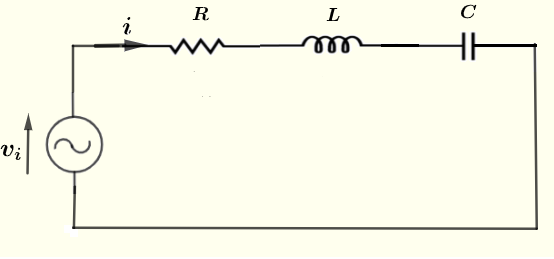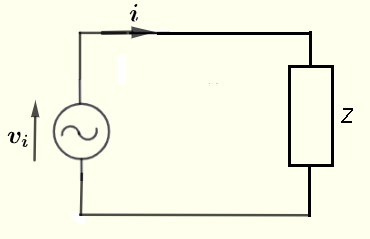# Power Calculator in Series RLC

  

A calculator to calculate the average power delivered to a resistor, a capacitor and an inductor in series, as shown below, is presented.The calculator gives the impedance of the series circuit as a complex numbers in standard form , its modulus and argument, the power factor and the average power.

## Formula for The Average Power Delivered to a series RLC CircuitThe formula of the average power delivered to an impedance $Z$ as shown in the circuit below is given by
$\displaystyle \quad \quad P_a = \dfrac{V_0^2}{2 |Z|} \cos \theta$
where $V_0$ is the peak voltage of the source voltage $v_ i$. $|Z|$ is the modulus of $Z$ and $\theta$ its argument.
The term $\cos \theta$ is called the power factor.

and define the following parameters used in the calculations
$\omega = 2 \pi f$ , angular frequency in rad/s
$X_C = 1 / (\omega C)$ , the capacitor reactance in ohms $(\Omega)$
$X_L = \omega L$ , the inductor reactance in ohms $(\Omega)$
The formula of the impedance $Z$ of the series RLC circuit shown above and write it in standard complex form as follows
$Z = R + (X_L - X_C) j$
and in polar form as follows
$Z = |Z| e^{j \theta}$
The formulae for the modulus $r$ and argument $\theta$ are given by (see proof at the bottom of the page)

Modulus: $|Z| = r = \sqrt {R^2 + (X_L - X_C)^2 }$ in ohms $(\Omega)$

Argument: $\theta = \arctan \left(\dfrac{X_L - X_C}{R} \right)$ in radians or degrees

## Use of the calculator

Enter the resistance, the capacitance, the inductance and the frequency as positive real numbers with the given units then press "calculate".

 Peak Voltage $V_0$ = 5 Volts Resistance R = 1000 mΩ Ω KΩ MΩ Capacitance C = 100 pF nF μF mF F Inductance L = 100 μH mH H Frequency f = 1.59154943092 GHz MHz kH Hz mHz